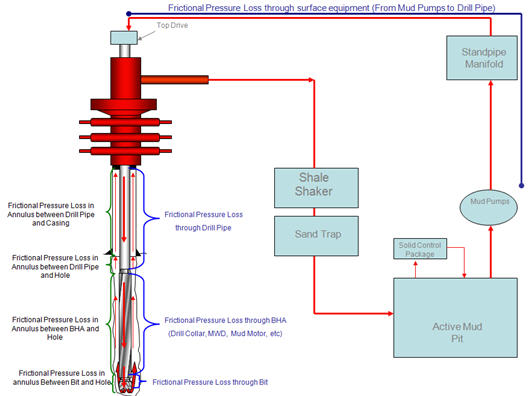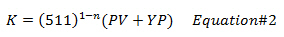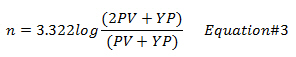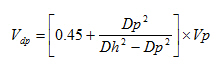## Introduction to Drilling Hydraulics

Drilling hydraulics affect directly drilling performance and this topic will focus on the basic principle of the drilling hydraulics.

Circulation System on Drilling Rigs

Typically, the rig circulation system is composed of mud pumps which deliver drilling mud from mud pits to hoses and pipes down to the bottom of the hole. Drilling mud is moved through a bit into the annulus and is finally returned back to solid control equipment and the mud pits (you can see from the following diagram).Frictional Pressure Loss

Frictional Pressure Loss is pressure loss created when drilling mud flows through a flow path. Frictional pressure loss affects on pump pressure because higher frictional pressure loss, higher pump pressure is required to maintain the pump rate.

The following parameters affecting frictional pressure loss are as follows:

• Inner diameter of drill pipe, drill collar and heavy weight drill pipe

• Down hole tool as a mud motor, a rotary steerible tool, a MWD/LWD, etc

• Length of drill pipe, drill collar and heavy weight drill pipe

• Drilling fluid properties – mud weight, mud rheology (PV/YP)

• Hole size

• Length of the well

• Inner diameter of the previous casing string

• Flow area of the bit

• Flow rate

For more understanding, the diagram below demonstrates the frictional pressure loss during drilling operation.## Cutting Carrying Index – Simple Tool To Determine Hole Cleaning

To get the good idea on how good of the hole cleaning is, the another method called Cutting Carrying Index (CCI) is utilized. The CCI is an empirical relationship from real data and the equation is below:

CCI = (K × AV × MW )÷(400,000) — Equation 1

Where;

AV is annular velocity in ft/min.

MW is mud weight in ppg.

K is a Power Law Constant.

The Power Law constant (K) can be calculated from the equation below:Where;

PV is plastic viscosity in centipoises.

YP is yield point in lb/100sqft

n is flow behavior index.

The flow behavior index (n) can be determined by the following equation:Where;

PV is plastic viscosity in centipoises.

YP is yield point in lb/100sqft

How will the CCI tell you about hole cleaning?

If CCI is equal to 0.5 or less, the hole cleaning is poor and the hole problem may be seen.

If CCI is equal to 1.0 or greater, it indicates that the hole cleaning is good.

## Determine surge and swab pressure method 2 Calculation Example

This section will show to how to use the formula from the previous topic, surge and swab pressure calculation 2nd method , to determine surge and swab pressure. I still use the same parameters as I used for the first method of surge and swab pressure calculation so you can compare the result between method#1 and method#2.

The well information is listed below:

θ300 = 85

θ600 = 130

Hole diameter = 9 inch

Drill Collar = 6.25 inch

ID of drill collar = 2.5 inch

Drillpipe diameter = 5 inch

ID of drill pipe = 3.5 inch

Average pipe running or pulling speed = 250 ft/min

Drill Pipe Length = 12000 ft

Drill Collar Length = 800 ft

Current Mud Weight = 12.5 ppg

Well TVD = 9000 ft

## Determine surge and swab pressure method 2

This is another method for calculating surge and swab pressure. With this method, there are 3 assumptions as follows:

• Closed ended pipe (plug flow)

• Laminar flow around drill pipe

• Turbulent flow around drill collar

How to determine surge and swab pressure with this method

1. Determine pressure around drill pipe

2. Determine pressure around drill collar

Note: The calculation methodology is different from the first method because both pressure losses are determined by separate set of equations.

3. Determine total pressure loss by summation of step 1 and step 2

4. Determine surge and swab pressure

### Determine pressure loss around drill pipe by following calculations

1. Determine fluid velocity around drill pipeWhere;

Vdp is the fluid velocity around drill pipe in ft/min.

Vp is pipe movement velocity in ft/min.

Dp is drill pipe diameter in inch.

Dh is hole diameter in inch.

## Determine surge and swab pressure for open-ended pipe

You’ve learn how to determine surge and swab pressure from the previous topic (Surge and swab pressure method 1) and this topic will demonstrate you how to use those equations to determine surge and swab pressure for the open-ended pipe.

Example

The well information is listed below:

θ300 = 85

θ600 = 130

Hole diameter = 9 inch

Drill Collar = 6.25 inch

ID of drill collar = 2.5 inch

Drillpipe diameter = 5 inch

ID of drill pipe = 3.5 inch

Average pipe running or pulling speed = 250 ft/min

Drill Pipe Length = 12000 ft

Drill Collar Length = 800 ft

Current Mud Weight = 12.5 ppg

Well TVD = 9000 ft